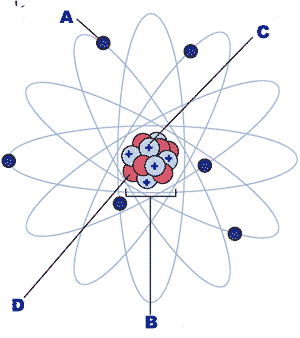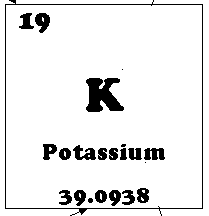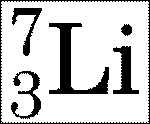Java Games: Flashcards, matching, concentration, and word search.# Science 9 General: Basic Chemistry (Atomic Structure)

AB
What are the building blocks of all matter?atoms
The nucleus of an atom is made up of ____ .protons and neutrons
Electrons have a ___ chargenegative
Protons have a ___ chargepositive
Neutrons have a ___ chargeneutral (no) charge
Which type of particle orbits the nucleus?electrons
Positive charges are attracted to ____ chargesnegative
Positive charges are repelled by ____ chargespositive
Negative charges are repelled by ____ chargesnegative
Which subatomic particle would an electron would be attracted to?proton
The large center region of an atom is called the ___ of the atomnucleus
Each element is different because they have a different number of ____.protons
All of the elements are listed in the ____.periodic table
The ____ number is equal to the number of protons in an atom.atomic number
The ____ number is equal to the number of protons + the number of neutrons.mass number
The mass number is equal to the number of ______.protons and neutrons
The atomic number is equal to the number of ____ in an atomprotons
The number below the chemical symbol on the periodic table that has a few decimal places is known as the ____.average atomic mass
If an element has a mass # of 23 and an atomic # of 11, how many protons will it have? Neutrons?11 protons and 12 neutrons
The _______ is equal to the number of subatomic particles in the nucleus.mass number
Protons, neutrons, and electrons are often referred to as ______ particles.subatomic
Each different element has a different number of _____ in their nucleus.protons
What is "A" pointing to?,an electron,What is "B" pointing to?,The nucleus,What is "C" pointing to?,proton,What is "D" pointing to?,neutron,What is smaller than an electron?Chuck Norris' sense of fear (but no one has been brave enough to discover it yet),Atoms of different elements have different numbers of __________.protons
Atoms of the same element always have ________ number of protonsthe same
The number 19 on the periodic table box below is the ____ of potassium.,atomic number,The number 39.0938 is the _____ of potassium.,average atomic mass (Remember, average atomic mass is the weighted average mass of all the isotopes of an element)
According to the image below, how many protons does potassium have?,19 (you can tell by the atomic number, which is 19),How many electrons would an atom of potassium have? (Hint: The atomic number is 19 and the average atomic mass is 39.10 amu's),19 (the same as the number of protons which is the same as the atomic number, because atoms are electrically neutral so you need the same number of negatively charged electrons as positively charged protons. The average atomic mass doesn't tell you any useful information about the number of electrons),The graphic below shows the nuclear symbol for an isotope of lithium. The superscript 7 refers to the ____ number while the subscript 3 refers to the ____ number.,The 7 is the mass number and the 3 is the atomic number,This activity was created by a Quia Web subscriber. Learn more about QuiaCreate your own activities